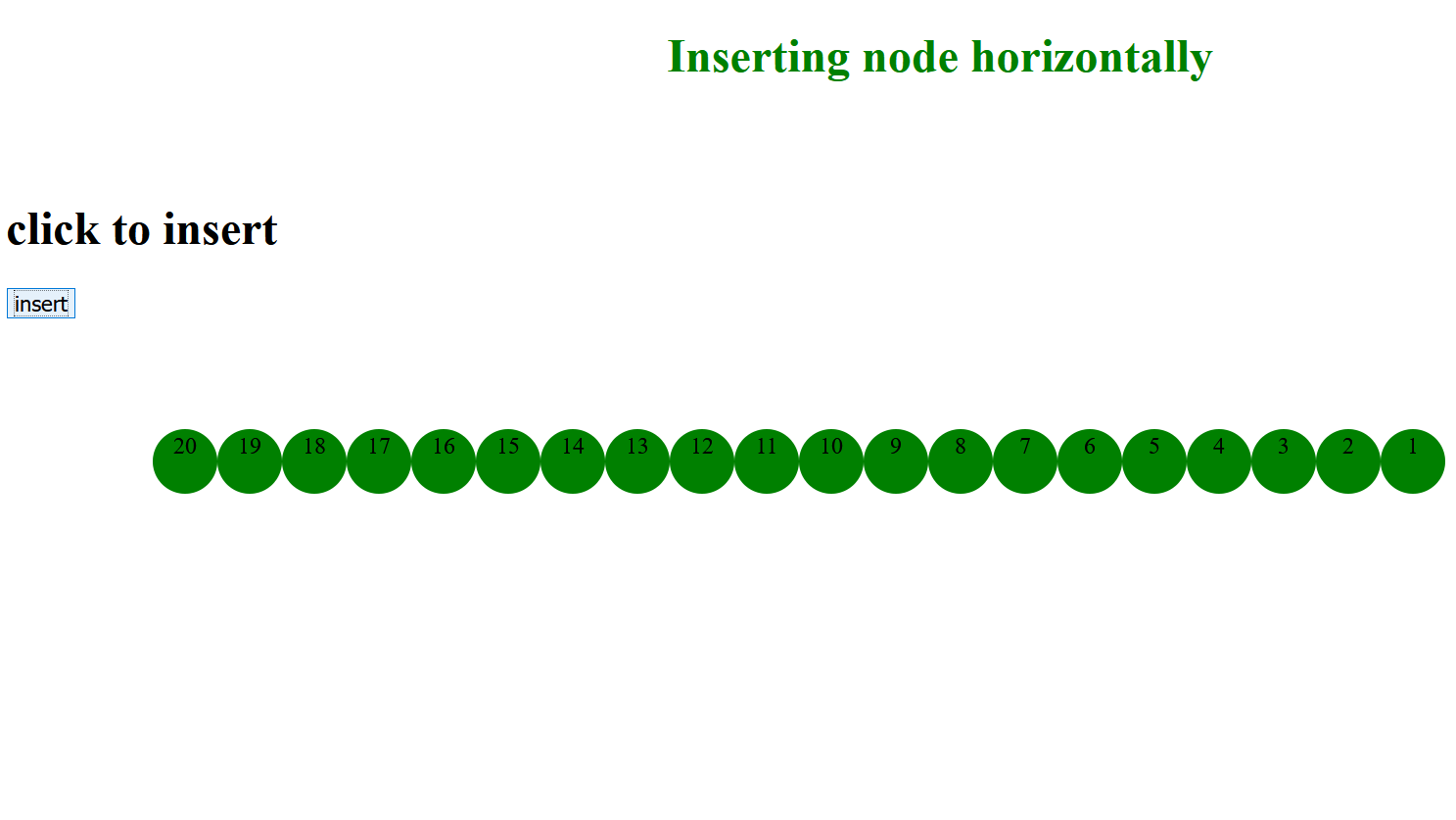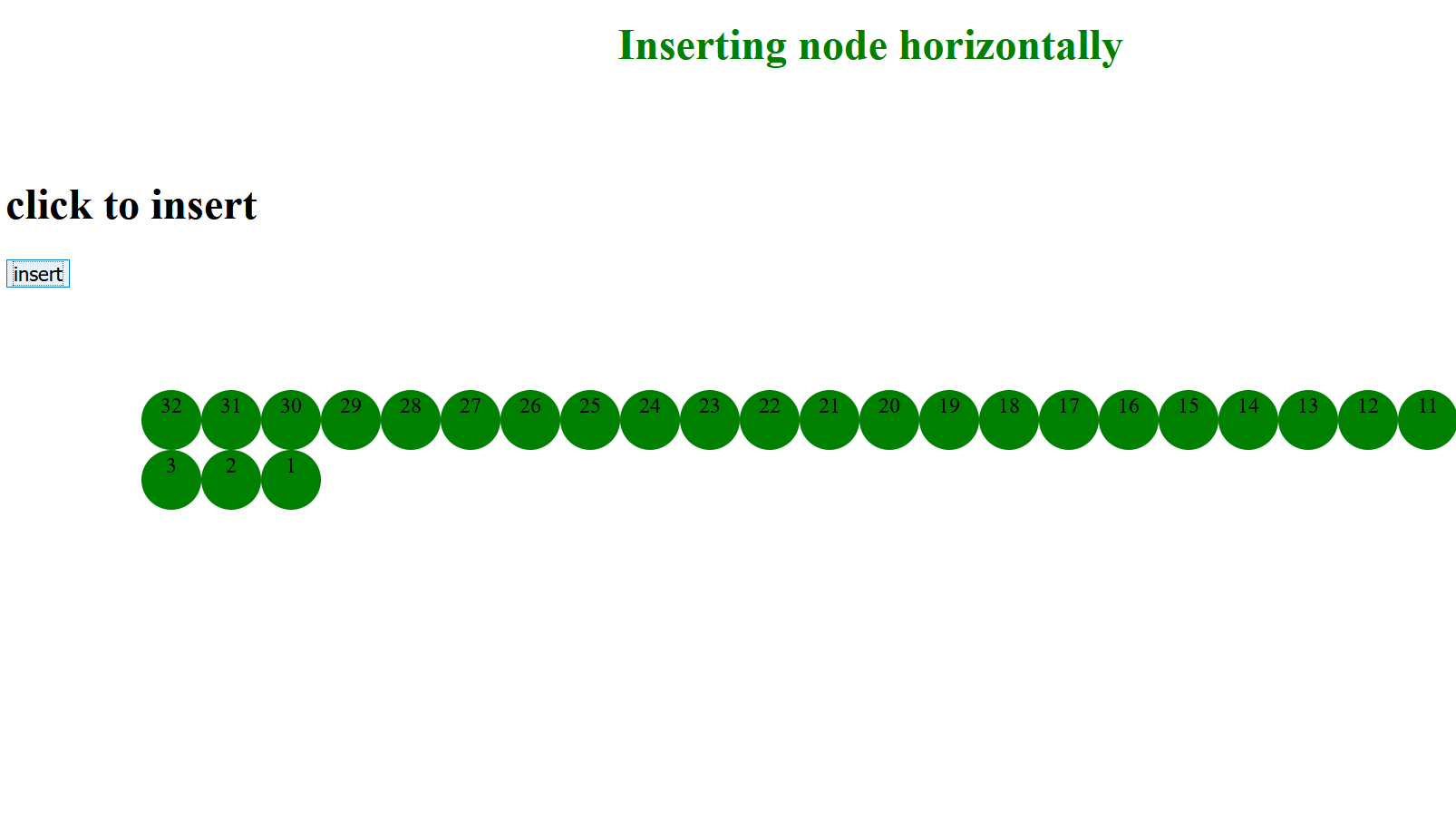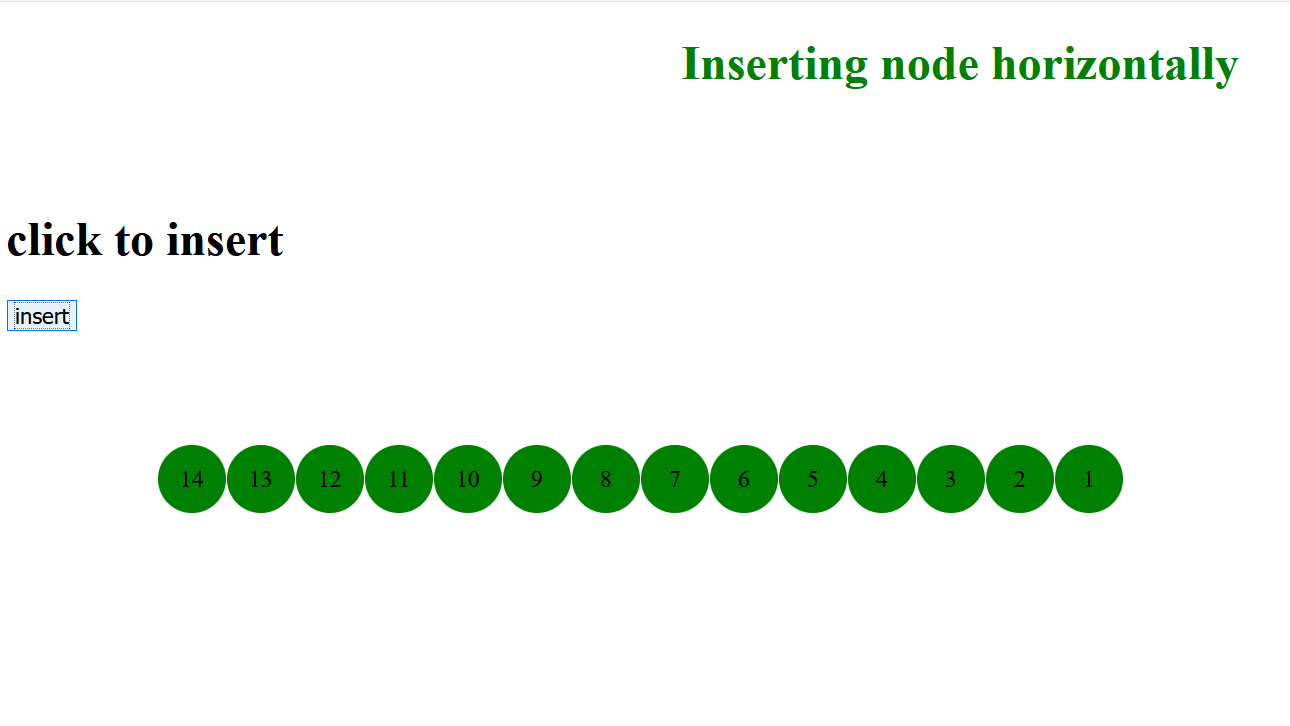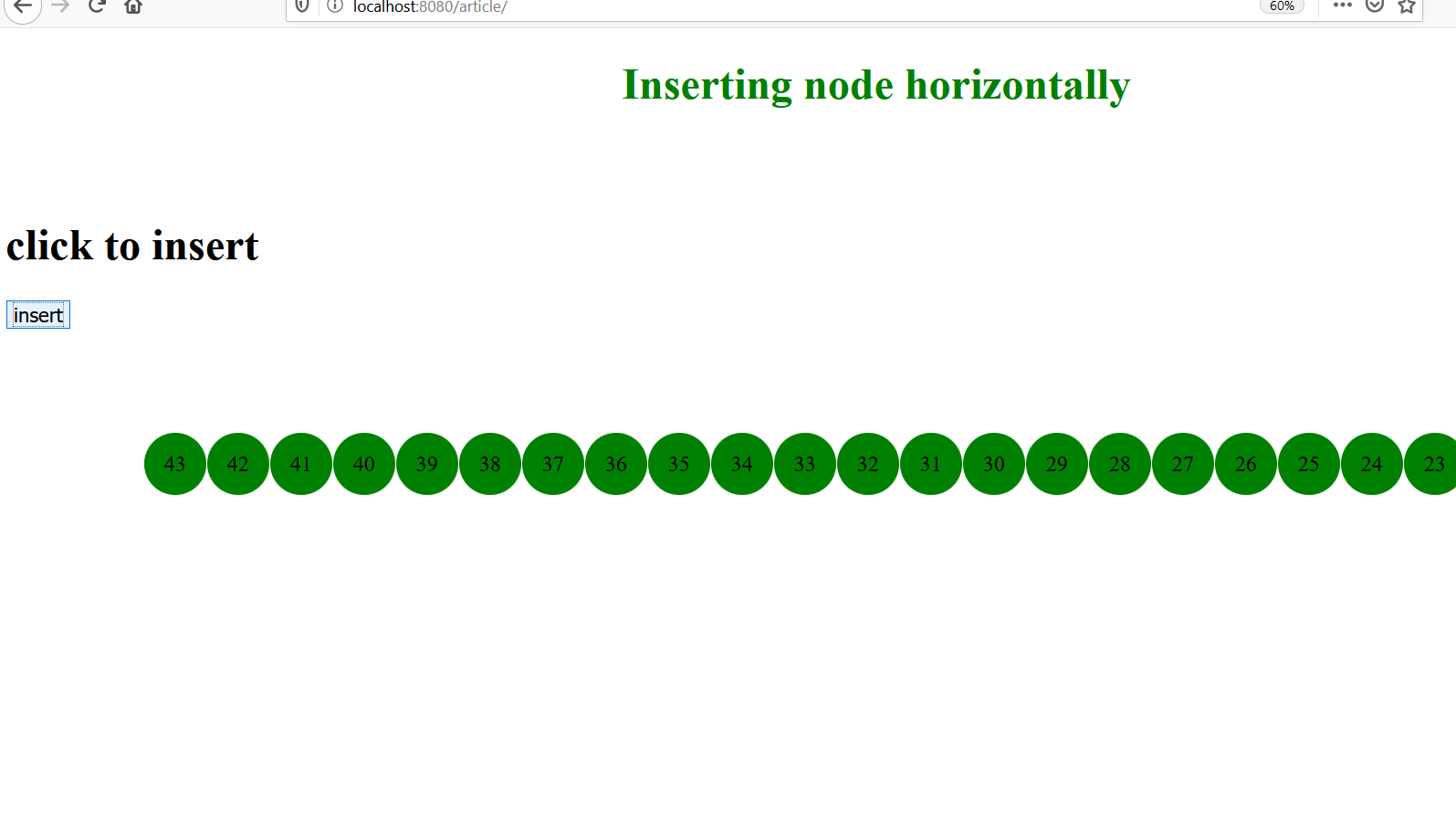Related Articles

# How to add an element horizontally in Html page using JavaScript?

• Last Updated : 22 Jun, 2020

There can be some cases where we have the requirement to add elements in a horizontal manner. Like, if your elements are linked list nodes and you want to add them horizontally.
Now the question arises how we can do this in better way.
One approach can be to use “display” property with the value “inline grid” as shown in the following program:
When the user clicks the button to add the node. The node starts to prepend in the linked list sequentially. To achieve this we have created the “div” with the “display” property whose value we have given as “inline-grid”.

Example-1

## javascript

 ```````````  ````   ``
``   ``

``          ``Inserting node  horizontally``   ``

``   ``
`` ` `

`` ` `

click to insert

`` ` `
`` ` ```
`` ` `
``    ``

`` ` `
``
``  ` ` `` ` ``` ` ``````

Output:Code explanation-
We have created a

## html

 `“<``div``>”`

element with id as “division” which will be our main division in which we add different “div” as node. In the “insert()” function we have created the.

## html

 `“<``div``>”`

which will be the element of our linked list we have added a “number” which will start from 1 and will increment as we add more elements in the list. For the node to be prepended horizontally we have used the display property with value ” inline-grid”.
But, there is a problem here in this solution, as we keep on adding elements in the list after a certain node the elements start to jump to the line which is not desired. This is what demonstrated in the output below.What if we want to add every node on the single line?
Another approach:
In this approach, to achieve the same thing we have used

## html

 `“<``table``>”.`

So, the idea is that in a table we can create one table row i.e.

## html

 `” <``tr``>”`

and for adding each node we wiil create table data i.e.

## html

 `”<``td``>”`

. So, there will be one “tr” and every node will be treated as

## html

 `“<``td``>”`

. So, in this way an infinite number of nodes can be added by using a table.
We can see its implementation through program.

Example- 2:

## javascript

 ````````````` ` `
``

``     ``Inserting node  horizontally``

``
``

`` ` `

click to insert

`` ` `
``
`` ` ```
``
`` ` `

`` ` ```
``
```````` ` ``` ` ``` ` ``` ` ``` ` ``

OutputAfter inserting many nodesMy Personal Notes arrow_drop_up